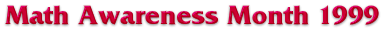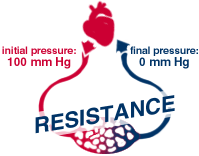```

```# Pressure and Blood Flow

It is clear that the higher the pressure exerted by the heart, the faster blood will flow. This is an example of a direct or proportional relationship between two quantities.There is also another factor which controls the blood flow rate, and it is the resistance of the blood vessels to blood flow. This resistance is simply due to the width 1 of the vessels - it's hard to push a lot of blood through a thin tube! Thus, we have an inverse relationship between blood vessel resistance and the blood flow rate - the higher the resistance, the slower the flow rate.

The relationship is expressed as follows:Now let's see if this makes any sense in numbers. The usual pressure difference between the left and right ventricles is about 100 mmHg. The normal cardiac output (the blood flow in the above equation) is about 5 liters/minute. The total peripheral resistance is about 20 (mmHg*min/liters).a) Resistance = 80
b) Resistance = 20
c) Resistance = 4
d) Resistance = 1

1 For a more detailed explanation, see the exhibit "Poiseuilles Law" at http://grad.math.arizona.edu/~walton/biomath/poiseuille1.htm

M & B Exhibits | intro | pg 2 | pg 3 | pg 4 | pg 5 | pg 6 | pg 7 | pg 8